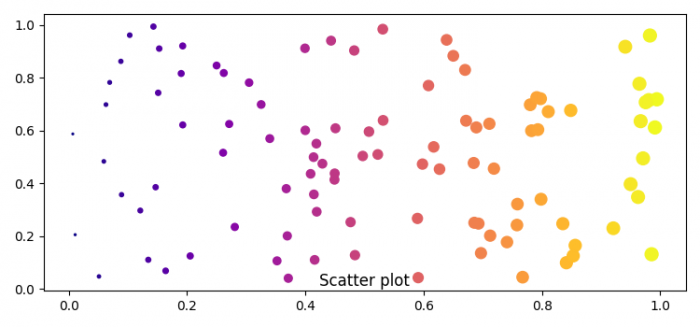# How to put the title at the bottom of a figure in Matplotlib?

To put the line title at the bottom of a figure in Matplotlib, we can take the following steps −

• Set the figure size and adjust the padding between and around the subplots.

• Initialize a variable, N, to get the number of sample data.

• Plot the x and y data points using scatter() method.

• Set the title at the bottom of the figure in matplotlib, with y=-0.01.

• To display the figure, use show() method.

## Example

import numpy as np
from matplotlib import pyplot as plt

plt.rcParams["figure.figsize"] = [7.50, 3.50]
plt.rcParams["figure.autolayout"] = True

N = 100
x = np.random.rand(N)
y = np.random.rand(N)

plt.scatter(x, y, c=x, s=x*100+1, cmap="plasma")

plt.title('Scatter plot', y=-0.01)

plt.show()

## Output